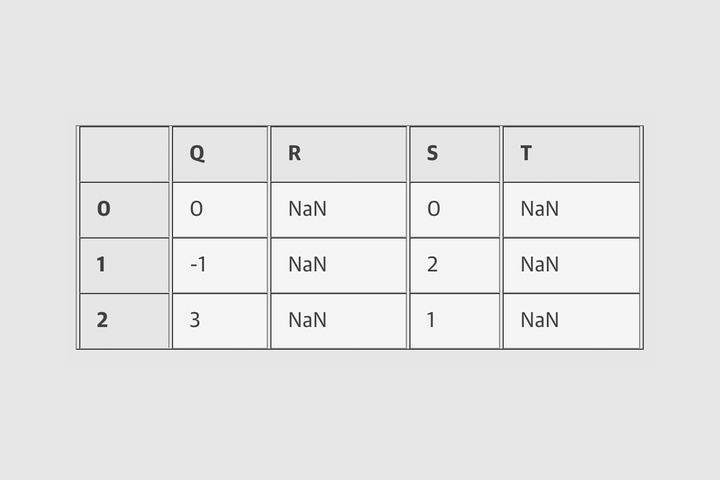Pandas中的运算
Python 数据科学手册(抢先版)

NumPy的重要特性之一是能够执行快速的元素级运算，既有基本的算术（加减乘除等），也有更为复杂的运算（三角函数、指数和对数等方法）。Pandas从NumPy继承了这些功能中的大部分，我们在第X.X节中介绍过的通用处理函数（universal functions简称ufuncs）是关键所在。

Pandas包含了一些有用的应变：对于像否运算以及三角函数这样的一元运算，这些通用方法在输出中会保留索引和列标签。而对于加法和乘法这样的二元运算来说，Pandas将会在对象传到方法中的时候自动对齐索引。这意味着保留数据的上下文以及组合来自不同源的数据—这两个在原始NumPy数组中容易出错的任务，在Pandas中几乎可以做到万无一失。此外，我们还将看到Pandas包含非常好的可以在一维Series结构以及二维DataFrame结构之间进行的运算。

Ufuncs：索引保留

```import pandas as pd
import numpy as np
```
```rng = np.random.RandomState(42)
ser = pd.Series(rng.randint(0, 10, 4))
ser
```
```df = pd.DataFrame(rng.randint(0, 10, (3, 4)),
columns=['A', 'B', 'C', 'D'])
df
```

```np.exp(ser)
```

```np.sin(df * np.pi / 4)
```

Ufuncs：索引对齐

Series中的索引对齐

```area = pd.Series({'Alaska': 1723337, 'Texas': 695662,
'California': 423967}, name='area')
population = pd.Series({'California': 38332521, 'Texas': 26448193,
'New York': 19651127}, name='population')
```

```population / area
```

```area.index | population.index
```

```A = pd.Series([2, 4, 6], index=[0, 1, 2])
B = pd.Series([1, 3, 5], index=[1, 2, 3])
A + B
```

```A.add(B, fill_value=0)
```

DataFrame中的索引对齐

```A = pd.DataFrame(rng.randint(0, 20, (2, 2)),
columns=list('AB'))
A
```
```B = pd.DataFrame(rng.randint(0, 10, (3, 3)),
columns=list('BAC'))

B
```
```A + B
```

```A.add(B, fill_value=np.mean(A.values))
```

Python运算和与它们等价的Pandas对象方法的表格如下：

 Operator Pandas Method(s) + add() – sub(), subtract() * mul(), multiply() / truediv(), div(), divide() // floordiv() % mod() ** pow()

Ufuncs：在DataFrame和Series之间的运算

```A = rng.randint(10, size=(3, 4))
A
```
```A - A
```

```df = pd.DataFrame(A, columns=list('QRST'))
df - df.iloc
```

```df.subtract(df['R'], axis=0)
```

```halfrow = df.iloc[0, ::2]
half row
```
```df – half row
```Pandas operations